# Experimental Investigation of Heat Transfer of Nanofluid in Elliptical and Circular Tubes

Experimental Investigation of Heat Transfer of Nanofluid in Elliptical and Circular Tubes

Ammar M. Al-TajerAbdulhassan A. Kramallah Ali M. Mohsen Nabeel Sameer Mahmoud

Air Conditioning and Refrigeration Techniques Engineering Department, University of Warith Al-Anbiyaa, Karbala 56001, Karbala-Baghdad Rd, Iraq

Corresponding Author Email:
ammar.altajer@uowa.edu.iq
Page:
665-671
|
DOI:
https://doi.org/10.18280/mmep.080420
10 November 2020
|
Revised:
2 April 2021
|
Accepted:
12 April 2021
|
Available online:
31 August 2021
| Citation

OPEN ACCESS

Abstract:

The paper presents experimental comparison of forced convection for steady state turbulent flow of nanofluid (Al2O3-distilled water) inside circular and elliptical (aspect ratio of 0.75) cross section tubes of identical circumference and tube surface area. Convection coefficient, pressure change, and fiction factor were compared at different Reynolds number (3,000-9,230) with different nanoparticles volume concentration (0.5%, 1.0%, and 1.5%). The results showed that Nusselt number increases with increasing Reynolds number and nanoparticle volume concentration. The pressure drops and friction factor of nanofluid are higher than the distilled water and are increasing as the volume concentration increases. Furthermore, the elliptical tube provided small increase in Nusselt number compared to that of circular cross sectional tube. However, the friction factor in the elliptical tube was slightly higher.

Keywords:

elliptical tube, Nusselt number, nanofluid, turbulent flow

1. Introduction

The recent technological advancement in electronic devices and data processing equipment has led to an urgent need for reliable and efficient cooling systems while keeping the size and weight as minimal as possible. Accordingly, numerous studies have been published proposing various techniques to increase the rate of heat transfer. For instance, using corrugated channels, rough tube walls and/or adding nanoparticles of high thermal conductivity to the conventional cooling fluids increasing the heat transfer rate [1-7].

Various experimental studies were published on nanofluid heat transfer behavior inside tubes of circular cross section with laminar forced convection flow [8-12]. Zanjani et al.  investigated the effect of graphene-water inside (4.2 mm) circular tube at Re= 1850, and their results showed that using only 0.02% graphene increased the thermal conductivity of the nanofluid by 10.3%. Gwon et al.  experimentally investigated the viscosity and pressure change of carbon nanofluid inside a 10.7 mm diameter circular tube. They observed that by inserting the nanoparticles, higher friction factor is obtained for laminar flow, while under turbulent conditions there was insignificant increase in friction factor. Yang et al.  studied experimentally the effect of graphite nanofluid flowing through circular tube and showed that Nusselt number is affected by size of nanoparticles, their temperature, particle loading and base fluid chemistry.

An experimental study using aqueous copper (Cu) nanofluid inside a circular tube was conducted by Wusiman et al.  to investigate the forced heat transfer characteristics for Reynold’s Number varying from 300 to 16000. For Re= 2000, using Cu/water increased the heat transfer coefficient by 62% at 1% volume fraction. Azmi et al.  investigated the effects of TiO2-water and SiO2-water flowing in horizontal circular tube with uniform heat flux on Nusselt number and pressure drop. The result showed that SiO2-water nanofluids provided maximum heat transfer enhancement of 33% at 3.0% volume concentration while the pressure drop was proportional to nanofluid density. Duangthongsuk et al.  studied the effect of TiO2-water nanofluid (volume concentration between 0.2% and 2.0%) in circular tube with turbulent flow conditions. In their study they showed that higher Nusselt number values are obtained when volume concentration of less than 1.0% is used.

One of the advantages of elliptical cross sectional tubes is that they have smaller resistance to the fluid than that of that of circular cross section tube, and this helps reducing the required power for pumping the fluid in the tube. Accordingly, elliptical tubes have become an increased interest by many researchers [18, 19]. Shariat et al.  performed three dimensional numerical study using Al2O3-Water nanofluid to investigate the laminar mixed convection flow inside elliptical tube. In their study, Nusselt number increased and the skin friction factor decreased with increasing nanoparticles volume fraction at a given Reynolds number. They also showed increasing the aspect ratio of the elliptic tube resulted in local skin friction factor reduction. Similarly, Hussein et al.  conducted a numerical investigation on the effect of elliptical tube on heat transfer and friction characteristics by using TiO2-water nanofluid with turbulent flow, and their data showed a 4% enhancement of friction factor and a 9% enhancement of Nusset number when compared to data of circular tube at similar flow Reynolds numbers.

From above literature and to the best of authors’ knowledge, there has been no experimental study investigating the effect of using Al2O3-distilled water nanofluid on forced heat transfer characteristics inside an elliptical tube. The present study is experimental investigation of nanofluid flow through elliptical and circular cross sectional tubes of similar tube perimeter and surface area. The effect of tube geometry (circular and elliptical), (Al2O3-distilled water) nanofluid volume fraction and flow Reynold’s number on average Nusselt number, friction factor and pressure drop are compared and discussed.

2. Experimental Procedure

2.1 Experimental facility

The experimental facility shown in Figure 1 consists of a test section, pump, reservoir tank, cooling system, flow meter, digital manometer, bypass and control box. This component setup is built as a closed-loop system. Two copper tubes, one of a circle cross section and the other is elliptical cross section, of 1 mm thickness and the total length of 2000 mm were used in the test section to study the effects of tube cross sectional geometry on the heat transfer performance as shown in Figure 2. The detailed dimensions of both tubes are listed in Table 1.

## 1.jpg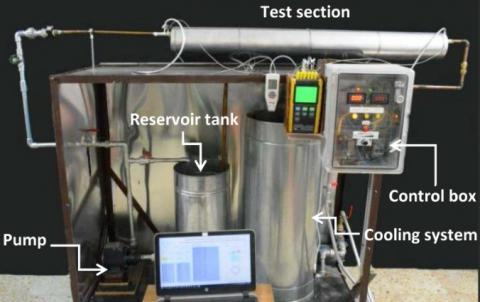Figure 1. The experimental facility

## 2.png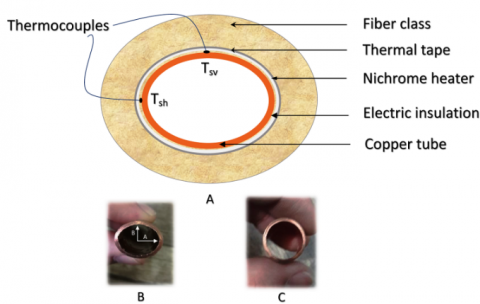Figure 2. (a) Schematic of the test section, (b) image of the elliptical tube, (c) image of the circular tube

Table 1. The elliptical and circular tube dimensions

 Parameter Elliptical tube Circular tube Internal radius A=8 mm, B= 6 mm 7 mm Aspect ratio B/A=0.75 B/A=1 Hydraulic dimeter 13 mm 14 mm Cross sectional area 150 mm2 156 mm2 Circumference 44 mm 44 mm Length 1300 mm 1300 mm Surface area 57856 mm 57856 mm

The bulk temperature is measured using two type-k thermocouples (model number TH-M6-K and measuring range of 0 to 400℃) installed at the inlet and outlet of the test section. For the circular tube five thermocouples (numbered 1 to 5 in Figure 3) were installed at distances of 150, 400, 650, 900 and 1150 mm from the tube inlet. For elliptical cross section tube, since there will be difference in temperature between the horizontal and vertical axis due to difference in transverse and conjugate diameters, another five thermocouples (numbered from 6 to 10 in Figure 3) are inserted in the horizontal plane at the distances of 150, 400, 650, 900 and 1150 mm from the inlet. Thus, the average temperature is considered at each distance from the vertical and horizontal thermocouple.

1500 Watt Nickel chrome AWG20 heater was used in order to obtain uniform heat flux boundary condition, and to minimize heat loss to the ambient, the test section was insulated with non-flammable blankest. Cooling system was used to reduce the nanofluid temperature after exiting the test section to ensure that the inlet flow temperature to the test section remains constant. Digital manometer is used to monitor the differential pressure.

## 3.pngFigure 3. Schematic of the test facility

2.2 Nanofluid preparation

Volume concentrations of (φ=0.5%, 1.0%, and 1.5%) were selected to prepare the Al2O3 - distilled water Nanofluid and to study the effect of using different concentrations on the average Nusselt number and pressure drop in the test section. Table 2 presents the distilled water and Al2O3 nanoparticles Thermo-physical properties.

Table 2. Thermo-physical properties at T=318 K

 Properties Water Al2O3 Density $\rho$ (kg/m3 ) 989 3900 Specific heat Cp (J/kg k) 4180.5 890 Thermal conductivity k (W/m.k) 0.6412 40

After preparing the nanofluid at specific volume concentration, its thermo-physical properties were measured using Hot Disk method and listed in Table 3. In order to determine its dynamic viscosity, capillary tube viscometer was used at different volume concentration, and to measure the density, pycnometer was used. All thermo-physical properties were obtained at temperature of 318 K.

Table 3. Thermo-physical properties of Al2O3 nanofluid at different volume concentrations T=318 K

 Properties Distilled water φ=0.5% φ=1.0% φ=1.5% Density (kg/m3) 989 1005 1009 1024 Specific heat (J/kg·k) 4180.5 4018 3790 3410 Thermal conductivity (W/m.k) 0.6412 0.659 0.6812 0.7097 Dynamic viscosity (kg/m·s) 566×10-6 973×10-6 977×10-6 992×10-6
2.3 Data analysis

Constant heat flux is applied on the elliptical and circular tubes (Figure 4) and the heat transfer coefficient and Nusselt number were calculated based on the following:

The local heat transfer coefficient equation for circular tube is :

$h(x)=\frac{\dot{q}}{T_{s}(x)-T_{m}(x)}$     (1)

where, q is the heat flux, Ts is the surface temperature and Tm is the bulk mean temperature. For elliptical tube in every section there are two thermocouples so we take average surface temperature in every section. Thus, for elliptical tube the local heat transfer coefficient is calculated from:

$h(x)=\frac{\dot{q}}{T_{s a v}(x)-T_{m}(x)}$     (2)

where, Tsav is the average surface temperature, which is given by:

$T_{s a v}(x)=\frac{T_{s h}(x)-T_{s v}(x)}{2}$     (3)

where, Tsh and Tsv are the horizontal and the vertical surface temperatures, respectively. The local Nusselt Number is found from:

$N u(x)=\frac{h_{x} D_{h}}{k}$     （4）

For thermal fully developed flow, the average Nusselt number is calculated from:

$N u=\frac{1}{L} \int_{0}^{L} N u(x) \cdot d x$    (5)

Simpson rule of 1/3 is used to calculate the integration. The experimental result then compared with theoretical calculations of Gnielinsk's correlation  of fully developed turbulent flow inside as follows:

$N u=\frac{(f / 8)(R e-1000) P r}{1+12.7(f / 8)^{1 / 2}\left(P r^{\frac{2}{3}}-1\right)}$     （6）

For 1.5≤Pr≤2000, 3000≤Re≤500000.

where: $f=(1.58 \ln R e-3.82)^{-2}$

The Reynold's number and Prandtl’s number were calculated from:

$R e=\frac{\rho u D_{h}}{\mu}$    (7)

$P r=\frac{C_{p} \mu}{k}$      (8)

The fiction factor was calculated by using Darcy equation :

$f=\frac{2 \times \Delta p \times D_{h}}{u^{2} \times L \times \rho}$      (9)

The friction factor of the present work was compared with Petukov's equation .

$f=(0.79 \times \ln (\mathrm{Re})-1.64)^{-2}$      （10）

For 3000≤ Re ≤5000000.

## 4.png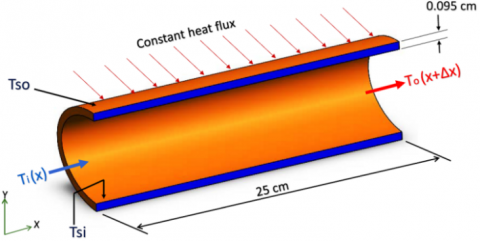Figure 4. Boundary conditions

3. Results and Discussion

In order to assess the accuracy of the experimental results, comparisons between the obtained data and Gnielinsk’s correlation and Petukov’s equation [21, 22] were carried out using distilled water for Nusselt number and friction factor at turbulent flow as shown in Figure 5, and the results showed good agreement.

Figure 6 shows the effect of nanofluid volume concentration on the average Nusselt number for circular and elliptical cross section tubes. The Nusselt number increases by increasing nanofluid volume concentration and the highest average Nusselt number of 116 was obtained at nanofluid volume concentration of φ=1.5% in the circular tube, while in the elliptical tube, the average Nusselt number was of about 120. However, further increase in Nusselt number can be obtained by increasing the volume concentration to an upper limit of φ=4% in water based Al2O3 nanofluids as reported by Sridhara et al. . Furthermore, increasing the nanofluid volume concertation showed a significant increase in the friction factor and pressure drop at lower Reynold’s number as shown in Figure 7 and Figure 8 respectively.

## 51.png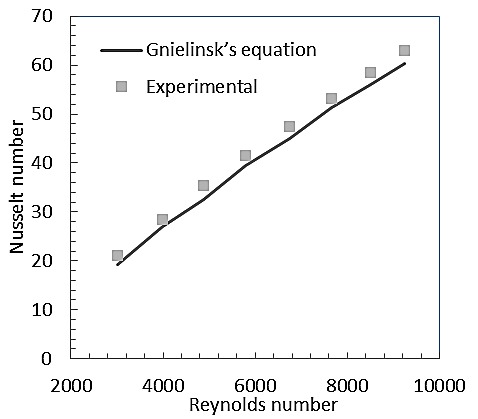(a) Nusselt number vs. Re

## 52.png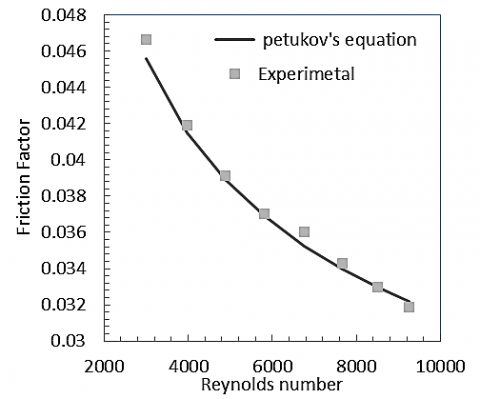(b) Friction factor vs. Re

Figure 5. Experimental results vs. Gnielinsk’s correlation and Petukov’s equation

## 61.png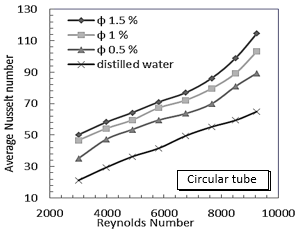## 62.png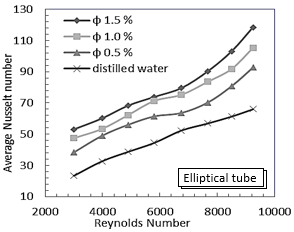Figure 6. Nusselt number vs. Re at different concentration

## 71.png## 72.pngFigure 7. Friction factor vs. Re at different concentration

## 81.png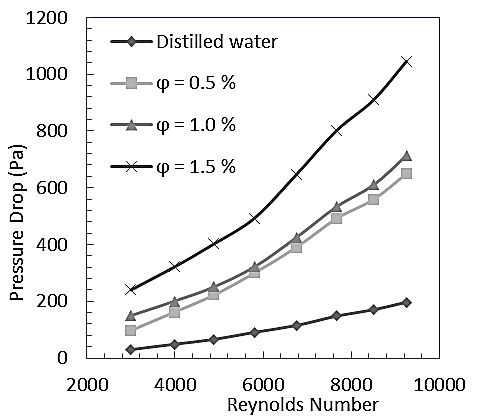(a) Circular Tube

## 82.png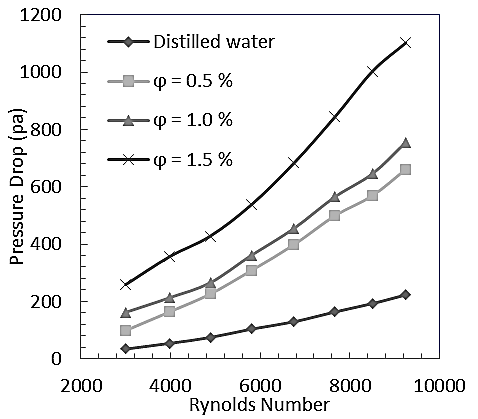(b) Elliptical Tube

Figure 8. Pressure drop vs. Re

## 9.pngFigure 9. Nusselt number in circular and elliptical tubes

## 10.png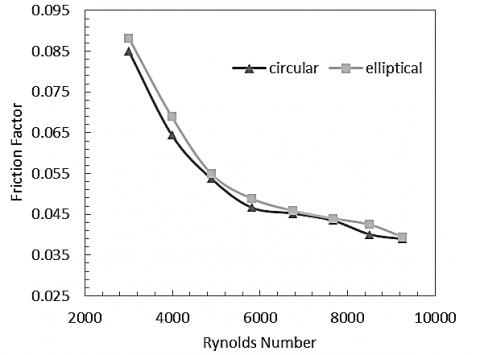Figure 10. Friction factor in circular and elliptical tubes

## 11.pngFigure 11. Pressure drop in circular and elliptical tubes

The effect of tube geometry (circular versus elliptical cross section) on Nusselt number, friction factor and pressure drop were studied for nanofluid volume concentration of φ=1.5% and Reynolds number ranging from 3,000 to 9,230 as shown in Figures 9 to 11 respectively. It can be noticed from Figure 9 that the elliptical cross section tube has highest average Nusselt number range as compared to the circular cross section tube, with maximum enhancement 5.7%. Furthermore, there is a slight increase in fiction factor due change in the cross sectional geometry as illustrated in Figure 10, which is caused by change in boundary layer form due to elliptic cross section shape. It is also noted that there is a small increase in pressure drop due to using elliptical tube at same volume concentration as shown in Figure 11.

The thermal efficiency is considered one of the most important relationships through which the efficiency of the pipes used in comparison as shown in the following relationship :

$\eta=\frac{\frac{N u_{n f}}{N u_{w}}}{\frac{f_{n f}}{f_{w}}}$      (11)

where, Nunf and Nuw are Nusselt number for nanofluid and distilled water respectively, fnf and fw are friction factor for nanofluid and distilled water respectively.

We can tell if the elliptical tube is more efficient for heat transfer systems compared to the circular tubes within the range of Reynolds number and nanofluid volume concentrations. In Figure 12, the thermal efficiency of the elliptical tube is higher than the circular tube at low Reynold’s number (of about 3000) since the Nusselt number in elliptical tube is higher. However, the efficiency decreases with increasing Reynolds number as the fiction factor increases (Eq. (11)) shows that the friction factor and efficiency are inversely proportional).

## 12.png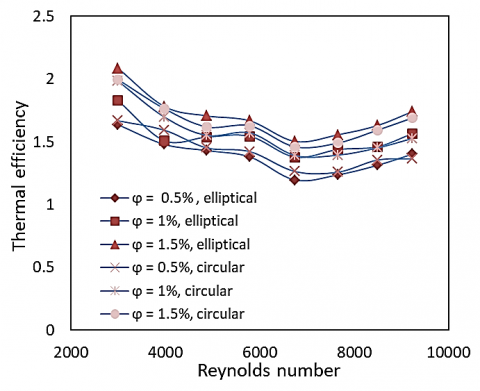Figure 12. Thermal efficiency

The logarithmic values of Nusselt number to friction factor relation have been depicted in Figure 13. This relation shows the effect of Nusselt number to friction factor of elliptical and circular cross-sectional tubes for different nanofluid volume concentrations and different Reynold’s number values. The Log (Nu/f) for distilled water for elliptical and circular tube increased with increasing Reynolds number since the Nusselt number increased and friction factor decreased. It is clearly shown that the elliptical tube introduces better heat transfer performance at low velocities. However, at higher flow velocities (Reynold’s number above 8000) the values of Log (Nu/f) are quite comparable, and this is due to the fact that the friction factor in the elliptical tube is increasing at the higher Reynold’s number and therefore increasing the obtained values of Log (Nu/f).

## 13.pngFigure 13. Log Nu/f vs. Re

4. Conclusions

The effects of tube cross sectional geometry on the heat transfer performance in circular and elliptical (aspect ratio of 0.75) cross sectional tubes of identical circumference and surface areas have been studied and compared in this paper. Nusselt number, pressure drop and friction factor were investigated using Nanofluid (Al2O3-distilled water) of different volume concentration with Reynold’s number ranging from 3,000 to 9,230. Fully developed turbulent flow conditions were applied at uniform heat flux. The result showed that heat transfer coefficient and Nusselt number increased when increasing the Reynold’s number and the nanoparticle volume concentration to 1.5%. Pressure drop and friction factor were also increased. Additionally, the elliptical tube showed small enhancement in heat transfer since the obtained Nusselt number was higher compared to that of circular cross sectional tube, while the pressure drop and friction factor in the elliptical tube were slightly higher.

Nomenclature
 AR aspect ratio A major radius of ellipse, m B minor radius of ellipse, m Tm mean bulk temperature, k Q heat flux, W/m2 p circumference, m ṁ mass flow rate, kg/s x,y,z coordinates C constant Cp specific heat, kJ/kg · k Ti inlet fluid temperature, k To outlet fluid temperature, k Ts surface temperature, k Tm bulk meat temperature, k L tube length, m Re Reynolds number Tsi inlet surface temperature, k Tso outlet surface temperature, k Nu Nusselt number Dh hydraulic diameter, m hx local heat transfer coefficient, W/m2 k k thermal conductivity, W/m.k Δp pressure drop, Pa U fluid velocity, m/s f friction factor Pr Prandtl number Greek symbols $\rho$ fluid density, kg/m3 η performance factor μ Dynamic viscosity, N.s/m2 φ nanofluid volume consternation
References

 Masoumi, N., Sohrabi, N., Behzadmehr, A. (2009). A new model for calculating the effective viscosity of nanofluids. Journal of Physics D: Applied Physics, 42(5): 1-6. https://doi.org/10.1088/0022-3727/42/5/055501

 Abdulkadhim, A., Abed, A.M., Mohsen, A.M., Al-Farhany, K. (2018). Effect of partially thermally active wall on natural convection in porous enclosure effect of partially thermally active wall on natural convection in porous enclosure. Mathematical Modelling of Engineering Problems, 5(4): 395-406. https://doi.org/10.18280/mmep.050417

 Radhi, D., Mohsen, A.M., Abdulkadhim, A. (2019). Experimental investigation of two-phase fluid flow over a rectangular obstructions located inside enlarged rectangular channel. Mathematical Modelling of Engineering Problems, 6(2): 183-187. https://doi.org/10.18280/mmep.060205

 Baheta A.T., Woldeyohannes, A.D. (2013). Effect of Particle size on effective thermal conductivity of nanofluiuds. Asian Journal of Scientific Research, 6(2): 339-345. https://doi.org/10.3923/ajsr.2013.339.345

 Xuan, Y., Li, Q. (2000). Heat transfer enhancement of nano fluids. International Journal of Heat and Fluid Flow, 21(1): 58-64. https://doi.org/10.1016/S0142-727X(99)00067-3

 Fotukian, S.M., Nasr, M. (2010). Experimental study of turbulent convective heat transfer and pressure drop of dilute CuO/water nanofluid inside a circular tube. International Communications in Heat and Mass Transfer, 37(2): 214-219. https://doi.org/10.1016/j.icheatmasstransfer.2009.10.003

 Duangthongsuk, W., Wongwises, S. (2008). Effect of thermophysical properties models on the predicting of the convective heat transfer coefficient for low concentration nanofluid. International Communications in Heat and Mass Transfer, 35(10): 1320-1326. https://doi.org/10.1016/j.icheatmasstransfer.2008.07.015

 Patel, H.E., Sundararajan, T., Das, S.K. (2010). An experimental investigation into the thermal conductivity enhancement in oxide and metallic nanofluids. Journal of Nanoparticle Research, 12(3): 1015-1031. https://doi.org/10.1007/s11051-009-9658-2

 Nassan, T.H., Heris, S.Z., Noie, S.H. (2010). A comparison of experimental heat transfer characteristics for Al2O3/water and CuO/water nanofluids in square cross-section duct. International Communications in Heat and Mass Transfer, 37(7): 924-928. https://doi.org/10.1016/j.icheatmasstransfer.2010.04.009

 Ko, G.H. (2007). An experimental study on the pressure drop of nanofluids containing carbon nanotubes in a horizontal tube. International Journal Heat Mass Transfer, 50(23): 4749-4753. https://doi.org/10.1016/j.ijheatmasstransfer.2007.03.029

 Chandrasekar, M., Suresh, S., Senthilkumar, T. (2012). Mechanisms proposed through experimental investigations on thermophysical properties and forced convective heat transfer characteristics of various nanofluids - A review. Renewable and Sustainable Energy Reviews, 16(6): 3917-3938. https://doi.org/10.1016/j.rser.2012.03.013

 Ho, C.J., Liu, W.K., Chang, Y.S., Lin, C. (2010). Natural convection heat transfer of alumina-water nanofluid in vertical square enclosures: An experimental study. International Journal of Thermal Science, 49(8): 1345-1353. https://doi.org/10.1016/j.ijthermalsci.2010.02.013

 Akhavan-Zanjani, H., Saffar-Avval, M., Mansourkiaei, M., Sharif, F., Ahadi, M. (2016). Experimental investigation of laminar forced convective heat transfer of Graphene-water nanofluid inside a circular tube. International Journal of Thermal Science, 100: 316-323. https://doi.org/10.1016/j.ijthermalsci.2015.10.003

 Yang, Y., Zhang, Z.G., Grulke, E.A., Anderson, W.B., Wu, G. (2005). Heat transfer properties of nanoparticle-in-fluid dispersions (nanofluids) in laminar flow. International Journal Heat Mass Transfer, 48(6): 1107-1116. https://doi.org/10.1016/j.ijheatmasstransfer.2004.09.038

 Wusiman, K. (2013). Heat transfer characteristics of nanofluid through circular tube. Journal of Central South University, 20(1): 142-148. https://doi.org/10.1007/s11771-013-1469-z

 Azmi, W.H., Sharma, K.V., Sarma, P.K., Mamat, R., Najafi, G. (2014). Heat transfer and friction factor of water based TiO2 and SiO2 nanofluids under turbulent flow in a tube. International Communications in Heat and Mass Transfer, 59: 30-38. https://doi.org/10.1016/j.icheatmasstransfer.2014.10.007

 Duangthongsuk, W., Wongwises, S. (2010). An experimental study on the heat transfer performance and pressure drop of TiO2-water nanofluids flowing under a turbulent flow regime. International Journal of Heat and Mass Transfer, 53(1-3): 334-344. https://doi.org/10.1016/j.ijheatmasstransfer.2009.09.024

 Dawood, H.K., Mohammed, H.A., Sidik, N.C., Munisamy, K.M. (2015). Numerical investigation on heat transfer and friction factor characteristics of laminar and turbulent flow in an elliptic annulus utilizing nanofluid. International Communications in Heat and Mass Transfer, 66: 148-157. https://doi.org/10.1016/j.icheatmasstransfer.2015.05.019

 Shariat, M., Akbarinia, A., Nezhad, A.H., Behzadmehr, A., Laur, R. (2011). Numerical study of two phase laminar mixed convection nanofluid in elliptic ducts. Applied Thermal Engineering, 31(14): 2348-2359. https://doi.org/10.1016/j.applthermaleng.2011.03.035

 Hussein, A.M., Bakar, R.A., Kadirgama, K., Sharma, K.V. (2016). Heat transfer enhancement with elliptical tube under turbulent flow TiO2-water nanofluid. Thermal Science, 20(1): 89-97. https://doi.org/10.2298/TSCI130204003H

 Syam, L., Singh, M.K. (2013). Convective heat transfer and friction factor correlations of nanofluid in a tube and with inserts: A review. Renewable and Sustainable Energy Reviews, 20: 23-35. https://doi.org/10.1016/j.rser.2012.11.041

 Abbasian, A., Amani, J. (2013). Experimental investigation of diameter effect on heat transfer performance and pressure drop of TiO2-water nanofluid. Experimental Thermal and Fluid Science, 44: 520-533. https://doi.org/10.1016/j.expthermflusci.2012.08.014

 Sridhara, V., Satapathy, L.N. (2011). Al2O3-based nanofluids: A review. Nanoscale Research Letters, 6(1): 1-16. https://doi.org/10.1186/1556-276X-6-456